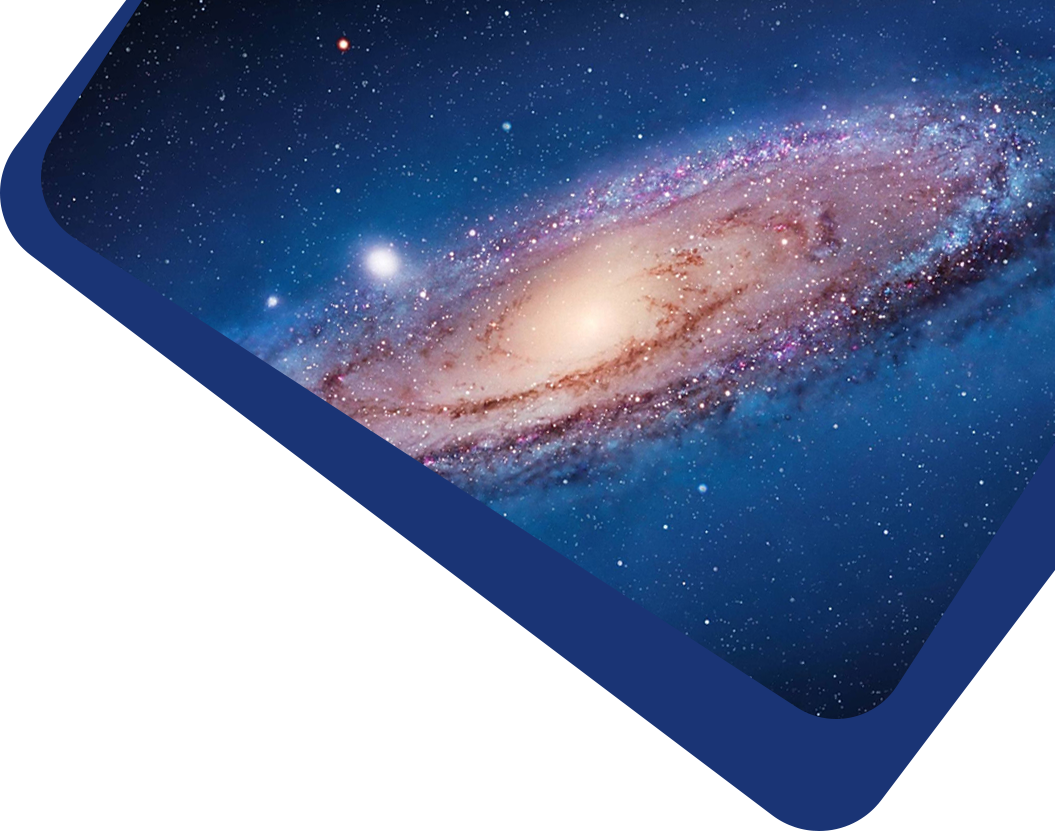Vol 22, No 5

## The First Photometric Study of AH Mic Contact Binary System

#### Atila Poro, M. G. Blackford, S. Ranjbar Salehian, E. Jahangiri, M. Samiei Dastjerdi, M. Gozarandi, R. Karimi, T. Madayen, E. Bakhshi, F. Hedayati

Abstract

Abstract The first multi-color light curve analysis of the AH Mic binary system is p_{\odot}sented. This system has very few past observations from the southern hemisphere. We extracted the minima times from the light curves based on the Markov Chain Monte Carlo (MCMC) approach and obtained a new ephemeris. To provide modern photometric light curve solutions, we used the Physics of Eclipsing Binaries (PHOEBE) software package and the MCMC approach. Light curve solutions yielded a system temperature ratio of 0.950, and we assumed a cold starspot for the hotter star based on the O’Connell effect. This analysis reveals that AH Mic is a W-subtype W UMa contact system with a fill-out factor of 21.3% and a mass ratio of 2.32. The absolute physical parameters of the components are estimated by using the Gaia Early Data Release 3 (EDR3) parallax method to be (M_h(M_{\odot})\) = 0.702(26), $$M_c(M_{\odot})$$ = 1.629(104), $$R_h(R_{\odot})$$ = 0.852(21), $$R_c(R_{\odot})$$ = 1.240(28), $$L_h(L_{\odot})$$= 0.618(3) and $$L_c(L_{\odot})$$ = 1.067(7). The orbital angular momentum of the AH Mic binary system was found to be 51.866(35). The components’ positions of this system are plotted in the Hertzsprung–Russell diagram.

Keywords

Keywords photometry – binary stars – individual (AH Mic)

Full Text
Refbacks

• There are currently no refbacks.